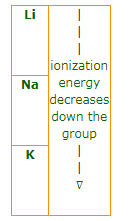# MCQ (Previous Year Questions) - Classification Of Elements & Periodicity In Properties (Level 2)

## 21 Questions MCQ Test Chemistry for JEE Advanced | MCQ (Previous Year Questions) - Classification Of Elements & Periodicity In Properties (Level 2)

Description
Attempt MCQ (Previous Year Questions) - Classification Of Elements & Periodicity In Properties (Level 2) | 21 questions in 42 minutes | Mock test for JEE preparation | Free important questions MCQ to study Chemistry for JEE Advanced for JEE Exam | Download free PDF with solutions
QUESTION: 1

### Moving from right to left in a periodic table, the atomic size is :               [JEE 1995]

Solution: When we moved left to right then size decreases because no of electron outermost orbital increased then size decrease question is right to left then answer is increase.
QUESTION: 2

### The increasing order of electronegativity in the following elements :  [JEE 1995]

Solution: Electronegativity increases from left to right
QUESTION: 3

### One element has atomic weight 39. Its electronic configuration is 1 s2, 2s2, 2p6, 3s2, 3p6, 4s1. The true statement for that element is : [JEE 1995]

Solution: Isotones are compound with same nutron number In the provided config and atomic mass and mass number difference is 20 same as in.
QUESTION: 4

An element belongs to period 2 and group 2 th number of valence electrons in the atoms of this element is.

Solution:
QUESTION: 5

The decreasing size of K+, Ca2+, Cl- & S2- follows the order :   [REE 1995]

Solution: More the number of electrons, bigger is the size. We know that negative sign means that electron has been gained or there are extra electrons present. So, size increases with increase in negative charge on the atom and size decreases with increase in the positive charge on the atom. S^ -2 has 2 more electrons and thus will be largest of them all. Similarly for Cl which will be greater than k+> Ca+2 thus, correct option is D.
QUESTION: 6

Which of the following oxide is neutral ?             [JEE 1996]

Solution: Carbon monoxide is slightly acidic in nature as it reacts with concentrated sodium hydroxide solution. But as it doesn't react with water it is considered as a neutral oxide.
QUESTION: 7

Which of the following has the maximum number of unpaired electrons

[JEE 1996]

Solution:
Fe^2+  has 1s^2,2s^2 2p^6,3s^2 3p^6 3d^6 configuration with 4 unpaired electron.
Hence (d) is the correct answer.
QUESTION: 8

The following acids have been arranged in the order of decreasing acid strength. Identify the correct order

[JEE 1996]

(I) ClOH

(II) BrOH

(III) IOH

Solution:
QUESTION: 9

The incorrect statement among the following is :                    [JEE 1997]

Solution:
(a) Correct statement : In a period, element of 2ndgroup ahs higher first ionization potential than element group of 13.
(b) Incorrect statement : Mg+ required less energy for further ionization than Na+ because of noble gas configuration of Na+.
(c) Correct statement : Ionization energy increases from left to right in a period.
(d) Correct statement : Mg2+ has noble gas configuration, require greater energy for further ionization than Na2+.
*Multiple options can be correct
QUESTION: 10

Which of the following are amphoteric ?

[REE 1997]

Solution:
QUESTION: 11

Identify the wrong sequence of the elements in a group

Solution:
QUESTION: 12

Anhydrous AlCl3 is covalent. From the data given below predict whether it would remain as a molecule or converts into ions in aqueous solution.                                                                        [JEE 1997]

[L.E. for AlCl3 = 5137 kJ/mol]

ΔH hydration for Al3+ = -4665 kJ/mol;

ΔHhydra for Cl- = -381 kJ/mol.

Solution:
*Multiple options can be correct
QUESTION: 13

Which one of the following statement (s) is (are) correct ?

Solution: Option A is correct because Cr gets stability due to half filled d and s orbitals , as we know that we get magnetic quantum numbers according to the value of azimuthal quantum number , for eg: if l(azimuthal) quantum number is 1 then magnetic quantum numbers will be -1,0,+1. We get C option correct by MOT. Hope you understand.
*Multiple options can be correct
QUESTION: 14

Assertion : F atom has less negative electron gain enthalpy than Cl atom                 [JEE 2000]

Reason : Additional electron is repelled more efficiently by 3p electron in Cl atom than by 2p electron in F atom.

Solution:
*Multiple options can be correct
QUESTION: 15

Assertion : Al(OH)3 is amphoteric in nature.

Reason : Al - O and O - H bonds can be broken with equal case in Al(OH)3                [JEE 2000]

Solution: Al(OH)3 forms water soluble complex Al(OH)4_ taking OH- from base OH- ions of Al(OH)3 reacts with H+ of acid to form H2O. so Al(OH)3 is amphoteric.
QUESTION: 16

The correct order of radii is :                                [JEE 2000]

Solution:
QUESTION: 17

The correct order of acidic strength is :                   [JEE 2000]

Solution:
QUESTION: 18

In the third period of the periodic table the element having smallest size is

Solution:
QUESTION: 19

The set representing correct order of IP1 is                [JEE 2001]

Solution: K > Na > Li : Incorrect, since ionization energy decreases down the group with increase in size. These elements belong to  same group (1st). The correct order of first ionization energy should be: K < Na < Li.Be > Mg > Ca : Correct. These are 2nd group
...

QUESTION: 20

Identify the least stable ion amongst the following :                      [JEE 2002]

Solution:
QUESTION: 21

Identify the correct order of acidic strengths of CO2, CuO, CaO, H2O :

[JEE 2002]

Solution:Use Code STAYHOME200 and get INR 200 additional OFF Use Coupon Code